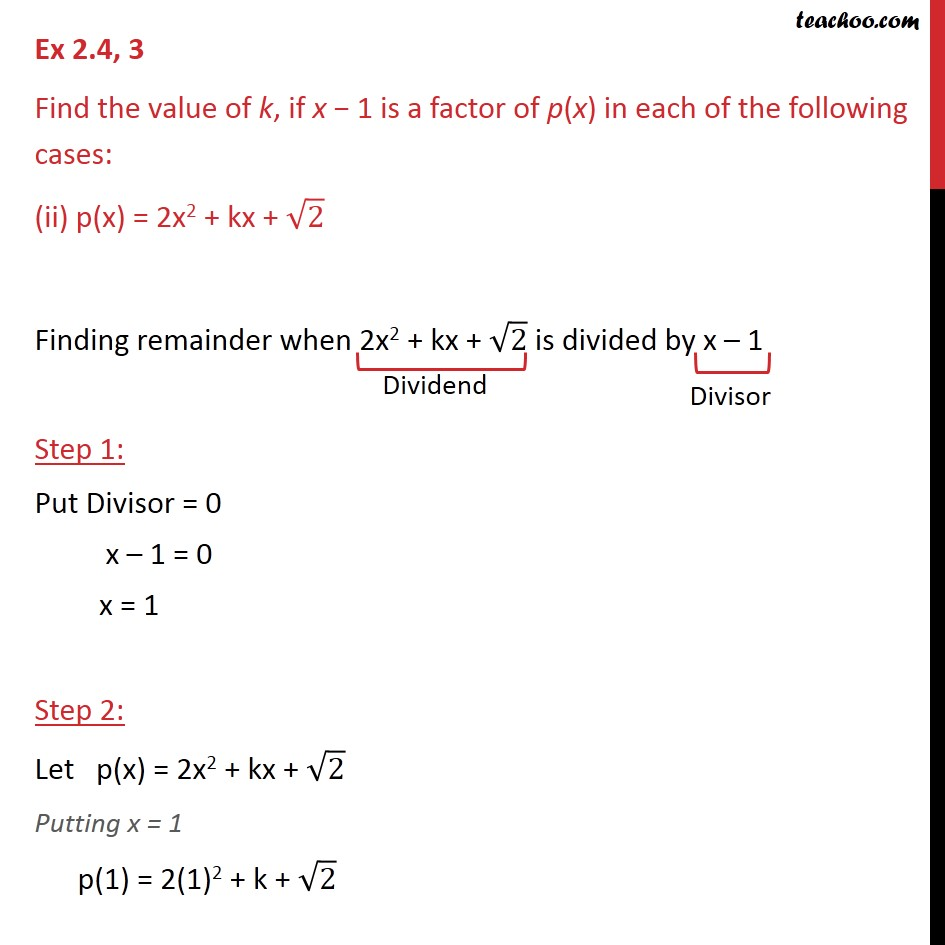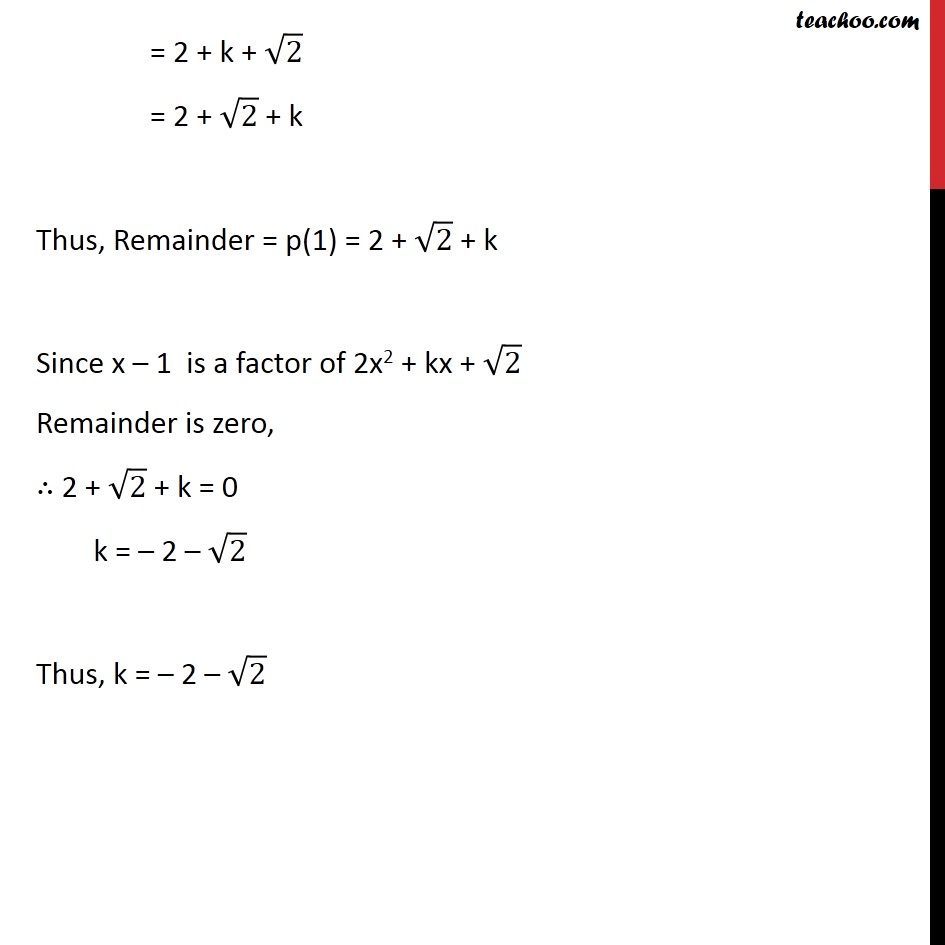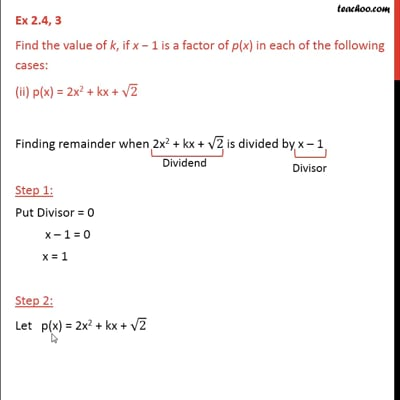Ex 2.4

Chapter 2 Class 9 Polynomials
Serial order wiseThis video is only available for Teachoo black users

Introducing your new favourite teacher - Teachoo Black, at only ₹83 per month

### Transcript

Ex 2.4, 3 Find the value of k, if x 1 is a factor of p(x) in each of the following cases: (ii) p(x) = 2x2 + kx + 2 Finding remainder when 2x2 + kx + 2 is divided by x 1 Step 1: Put Divisor = 0 x 1 = 0 x = 1 Step 2: Let p(x) = 2x2 + kx + 2 Putting x = 1 p(1) = 2(1)2 + k + 2 = 2 + k + 2 = 2 + 2 + k Thus, Remainder = p(1) = 2 + 2 + k Since x 1 is a factor of 2x2 + kx + 2 Remainder is zero, 2 + 2 + k = 0 k = 2 2 Thus, k = 2 2